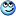# Ampere's law for a larger diameter?

PhiowPhi
Most of the problems I've worked on involve wires of small radii, however, if DC current flows within large radii of conductive wires, would I need to use current density ##J## for calculating the magnetic field at a point ## r > R## instead of ##I## if it we're known?

With relevance to a wires with large radii, would ampere's law change? Or still valid regardless of large or small radii? Also, in relation to that matter, does the Lorentz force alter with the change of geometry of the wire(e.g large radius, shorter lengths etc...)?

Last edited:

PhiowPhi
I'm not sure as to when current density ##J## might be used in calculating the magnetic field and Lorentz force acting on a wire placed in a magnetic field. If a clarification of that could be posted would greatly help.

Mentor
Most of the problems I've worked on involve wires of small radii, however, if DC current flows within large radii of conductive wires, would I need to use current density JJ for calculating the magnetic field at a point r>R r > R instead of II if it we're known?
(I'm assuming R is the radius of the wire)

If the current density J is distributed with cylindrical symmetry around the axis of the wire, then you can use the total current I to calculate the magnetic field outside the wire. That is, you can pretend that the total current is "collapsed" into a line along the central axis of the wire.

This is analogous to the situation where you want to find the electric field outside a charge distribution: if the charge density ρ has spherical symmetry, you can pretend that the total charge is "collapsed" into a point at the center.

•PhiowPhi
PhiowPhi
Well, if the wire is carrying DC isn't it intuitive to assume current is uniformly distributed? Therefore, using ##I## would suffice for these types of problems?

Mentor
I've done and assigned exercises in which the current distribution is not uniform, but is still cylindrically symmetric. I just wanted to touch all the bases, because around here, if I don't, someone will probably come along and do it for me.